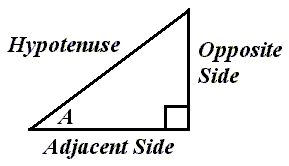# The Right Triangle and the Cotangent FunctionRelative to angle A, this is how the sides of a right triangle would be labeled.

The cotangent of angle A equals the length of the adjacent side divided by the length of the opposite side.

We could write: